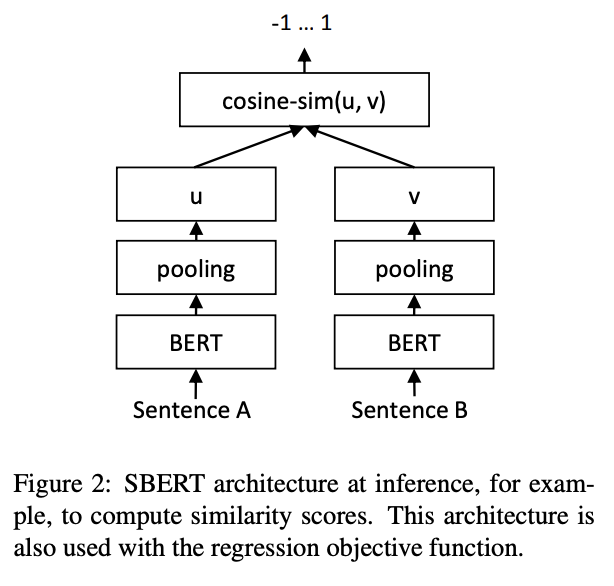#LiYuan /Amazon-Cup-Cross-Encoder-Regression

This model is actually very accurate for this rerank products given one query, intuitively inspired by information retrieval techniques. In 2019, Nils Reimers and Iryna Gurevych introduced a new transformers model called Sentence-BERT, Sentence Embeddings using Siamese BERT-Networks. The model is introduced by this paper https://doi.org/10.48550/arxiv.1908.10084.

This new Sentence-BERT model is modified on the BERT model by adding a pooling operation to the output of BERT model. In such a way, it can output a fixed size of the sentence embedding to calculate cosine similarity, and so on. To obtain a meaningful sentence embedding in a sentence vector space where similar or pairwise sentence embedding are close, they created a triplet network to modify the BERT model as the architecture below figure.from transformers import AutoTokenizer, AutoModelForSequenceClassification

tokenizer = AutoTokenizer.from_pretrained("LiYuan/Amazon-Cup-Cross-Encoder-Regression")

model = AutoModelForSequenceClassification.from_pretrained("LiYuan/Amazon-Cup-Cross-Encoder-Regression")


As we can observe from above figure, a pooling layer is added on the top of each BERT Model to obtain the sentence embedding $u$ and $v$. Finally, the cosine similarity between $u$ and $v$ can be computed to compare with the true score or make them semantically meaningful, then the mean square error loss, which is the objective function, can be backpropagated through this BERT network model to update the weights.

In our amazon case, the query is sentence A and concatenated product attributes are sentence B. We also stratified split the merged set into 571,223 rows for training, 500 rows for validation, 3,000 rows for test. We limited the output score between 0 and 1. The following scores represent the degree of relevance between the query and the product attributes in light of Amazon KDD Cup website; however, this can be adjusted to improve the model performance.

• 1: exact
• 0.1: substitute
• 0.01: complement
• 0: irrelevance

For this regression model, we used Pearson correlation coefficient and Spearman's rank correlation coefficient} to measure the model performance. If the correlation coefficient is high, the model performs well. The validation Pearson is \textbf{0.5670} and validation Spearman is \textbf{0.5662}. This is not bad result.

We also evaluated the model on the test set. We got 0.5321 for Pearson and 0.5276 for Spearman. These results from the test evaluation have results similar to those of the validation set, suggesting that the model has a good generalization.

Finally, once we have this fine-tuned Cross-Encoder Regression model, given a new query and its matched product list, we can feed them into this model to get the output score to rerank them so that this can improve the customer online shopping experience.